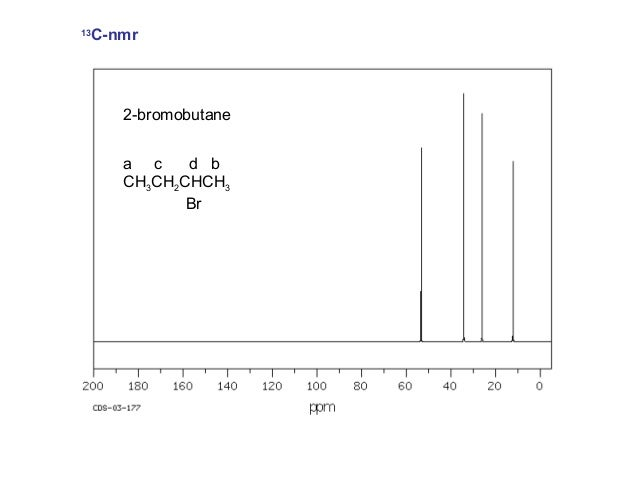# How is the peak affected in C^13 NMR by the number of H's attached to the carbon?

Jun 6, 2015

In a "normal" $\text{^13"C}$ NMR spectrum, the number of $\text{H}$ atoms attached to the carbon does not affect the peak.

That's because most $\text{^13"C}$ NMR spectra are broadband decoupled .

The couplings are removed by applying a continuous second radio signal that excites all the protons and cancels out their couplings with the $\text{C}$ atoms.

Thus, the spectrum of 2-bromobutane consists of four singlets.However, there is a technique called off-resonance decoupling , in which only the protons bonded to a given carbon atom split its signal.

Thus, a ${\text{CH}}_{3}$ group appears as a quartet, a ${\text{CH}}_{2}$ group appears as a triplet, a $\text{CH}$ group appears as a doublet, and a quaternary $\text{C}$ appears as a singlet.

So, the off-resonance decoupled spectrum of 2-bromobutane consists of two quartets, a triplet, and a doublet.